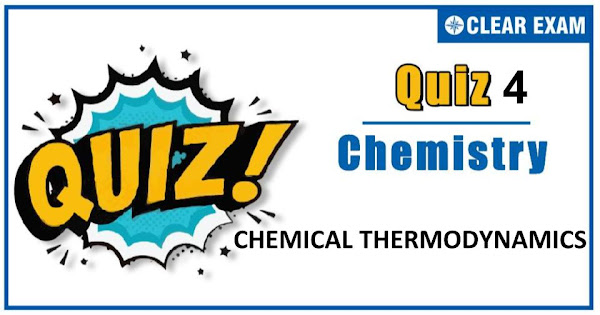## CHEMICAL THERMODYNAMICS QUIZ-4

The one subject in NEET which is candidates who can easily attain good marks is Chemistry. That's the reason, often, one doesn’t pay notice and choose to compromise it. But if one wants to rank above others, the tip is to be thorough with NEET chemistry concepts. The understanding of reactions and definite basic understanding is what requires major attention in Chemistry but once done it only gets simpler from there. The main focus on the to-do list should be on getting a hang of the NCERT syllabus of NEET chemistry..

Q1. Calorific value of carbohydrates is approximately:
•  4.0 kcal/g
•  16.0 kcal/g
•  20 kcal/g
•  9.0 kcal/g
Solution
a) Fuel value per g of substance produced on burning
Q2.Heat given to a system under isochoric process is equal to:
•  W
•  qp
•  ∆U
•  ∆H
Solution
c) For an isochoric process V= constant ; thus ∆V=0 from 1st law q=∆U-W or qv=∆U
Q3.  An engine operating between 150℃ and 25℃ takes 500 J heat from a higher temperature reservoir
if there are no frictional losses, then work done by engine is
•   165.85 J
•  169.95 J
•  157.75 J
•  147.7 J
Solution
d) T_2=150+273=423 K, T_1=25+273=298 K Q=500 K W/Q=(T2-T1)/T2 ∴W=500 ((423-298)/423)=147.7 J

Q4. The work done during the expansion of a gas from a volume of 4 dm3 to 6 dm3 against
a constant external pressure of 3 atm is
•  -6 J
•  -608 J
•  +304 J
•  -304 J
Solution
b) Work done(W)=-pext (V2-V1) =-3×(6-4)=-6 L atm = -6×101.32 J(∴ 1 L atm=101.32 J) = -607.92≈-608 J
Q5.If the enthalpy change for the transition of liquid water to steam is 30 kJ mol-1 at 27°C,
the entropy change for the process would be:
•  100 J mol-1K-1
•  10J mol-1K-1
•  1.0J mol-1K-1
•  0.1J mol-1K-1
Solution
a) ∆S=∆H/T=(30×103)/300=100 J mol-1 K-1
Q6. The ∆G in the process of melting of ice at -15℃ is:
•  ∆G=-ve
•  ∆G=+ve
•   ∆G=0
•  All of these
Solution
b) The melting of ice at -15℃ is not an spontaneous process
Q7. The gas in a refrigerator causes cooling on expansion because:
•  Work done by the gas is converted into heat
•  Heat of the gas is lost as work is done by the gas
•  The heat is spread over a large space
•  None of the above
Solution
b) The gives rise to cooling.
Q8.Heat of reaction at constant volume is equal to :
•  ƩUP-ƩUR
•  ƩUR-ƩUP
•  ƩHP-ƩHR
•  ƩHR-ƩHP
Solution
a) At constant volume, heat of reaction is ∆U.
Q9. Which phenomena cannot be described as oxidation?
•  Oxidation of coal in air
•  Burning of magnesium in nitrogen
•  Reaction of antimony with chlorine
•  Lighting of an electric lamp
Solution
d) It is a physical change.
Q10. Combustion of glucose takes place according to the equation
C6H12O6+CO2→6CO2+6H2O;∆H=-72kcal. How much energy will be required
for the production of 1.6 g of glucose (Molecular mass of glucose = 180)?
•  0.064 kcal
•  0.64 kcal
•  6.4 kcal
•  64 kcal
Solution
b) ∆H per 1.6 g =(72×1.6)/180=0.64 kcal#### Written by: AUTHORNAME

AUTHORDESCRIPTION## Want to know more

Please fill in the details below:

## Latest NEET Articles\$type=three\$c=3\$author=hide\$comment=hide\$rm=hide\$date=hide\$snippet=hide

Name

ltr
item
BEST NEET COACHING CENTER | BEST IIT JEE COACHING INSTITUTE | BEST NEET, IIT JEE COACHING INSTITUTE: Chemical Thermodynamics Quiz-4
Chemical Thermodynamics Quiz-4
https://1.bp.blogspot.com/-OUHYw2Lgmc4/X56kQ6U5R0I/AAAAAAAAIOQ/a2QMpxW27Asoy76AWymXFXTyAvPjwcI-gCLcBGAsYHQ/s600/Quiz%2BImage%2B20%2B%25282%2529.jpg
https://1.bp.blogspot.com/-OUHYw2Lgmc4/X56kQ6U5R0I/AAAAAAAAIOQ/a2QMpxW27Asoy76AWymXFXTyAvPjwcI-gCLcBGAsYHQ/s72-c/Quiz%2BImage%2B20%2B%25282%2529.jpg
BEST NEET COACHING CENTER | BEST IIT JEE COACHING INSTITUTE | BEST NEET, IIT JEE COACHING INSTITUTE
https://www.cleariitmedical.com/2020/11/quiz3.html
https://www.cleariitmedical.com/
https://www.cleariitmedical.com/
https://www.cleariitmedical.com/2020/11/quiz3.html
true
7783647550433378923
UTF-8